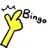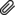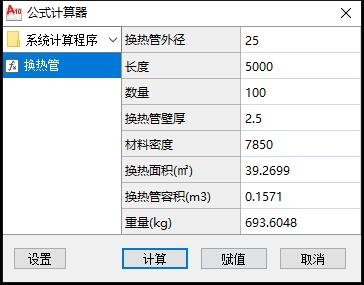# 公式计算器/函数计算器 JS

【公式计算器/函数计算器】

1 . 数学计算:

2 . 可用函数:
PI(), sin(n), cos(n), tan(n), sqrt(n), asin(n), acos(n), atan(n), lg(n), ln(n), exp(n), abs(n), ceil(n), floor(n), ^n

3 . 模版文件配置说明
3.1 模版存放目录为: “自定义目录\Calcs\分类目录\”, (必须在Calcs目录下面建子目录作为分类)
3.2 模版的扩展名为: *.calc
3.3 每一行设定一个变量, 每一行中包含4个区块, 分别以冒号和等号分隔, 如:
\$变量名:显示名称=值:下拉列表项(如果有)

``````   各个区块说明如下:
*** 变量名:     变量名以\$开头, 名称由字母、数字、下划线组成, 且首字符不能为数字
*** 显示名称:   显示在对话中
*** 值:         变量的默认值, 可以为空
*** 下拉列表项: 如果设置该内容(以分号分隔), 则该输入框可以通过下拉列表框选取  ``````

4 . 以下是一个完整的模版文件, 用于计算换热管的换热面积、管内容积、重量.

``````\$d:换热管外径=:19;25;32;38;45;57
\$t:换热管壁厚=:2.5;3;3.5
\$L:长度
\$n:数量=1
\$m:材料密度=7850:7850;7930
\$S:换热面积(㎡)=PI()*\$d*\$L*\$n*10^-6
\$V:换热管容积(m3)=PI()*(\$d*0.5-\$t)^2*\$L*\$n*10^-9
\$W:重量(kg)=PI()*(\$d-\$t)*\$t*\$L*\$n*10^-9*\$m  ``````

#### 样例下载换热管.calc (237B, 下载次数:12)